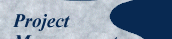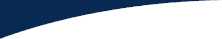Published here December 2004.
Explanatory Preamble | Brenda Originally WroteLouise Responds | Frank Chips In | Kathy … | Quentin RespondsBrenda Tries to Summarize | Max Comments | Glen Responds

Thank you for asking me. I have spotted this apparent "flaw" in the past and I do clarify the situation in my classes. It is not really a flaw at all, just a misunderstanding in the way the calculations are applied.

I first started using earned value analysis on real projects long before PMI ever coined the term "Planned Value". It was always BCWS, Budgeted Cost of Work Scheduled. This term may have been confusing for some people, which may be the reason PMI chose another term. It helps to be able to use BCWS and differentiate it from Total Budget at Completion (BAC).

In the example below, the "Planned Value" is used to mean two different things, hence the formulas will not give the correct answers for SV or SPI. It appears that the example is using PV to mean both the value planned to be spent at a certain point in time and the total budget to be spent by the time the work is completed. This is not the correct approach.

The earned value should be calculated as the Budget at Completion (could also be called Total Budget) for the work multiplied by the percent of work complete.

EV = BAC x % complete

The schedule variance should be calculated as the difference between this earned value and the budgeted cost of work scheduled up to that point in time, regardless of whether you choose to call this value PV or BCWS or anything else.

SV = EV - PV

Similarly, the SPI is calculated using the earned value and the budgeted cost of work scheduled up to that point in time.

SPI = EV/PV

The problem in the example below is that the initial calculation of earned value should use the total budget of 10,000 times the percent complete of 70%:

EV = 10,000 x 0.7 = 7,000

Then SV = EV - PV = 7,000 - 7,000 = 0, as expected.

I do encounter this difficult often and I have some good example problems that help to clarify it. Please let me know if you would like additional information or examples.

Louise Novakowski